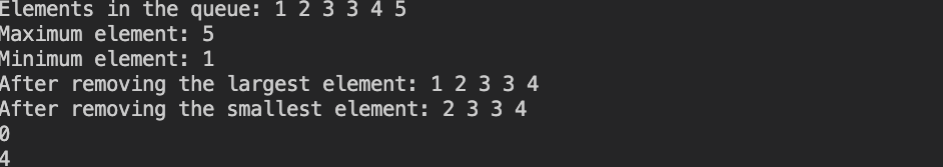# Double Ended Priority Queue

## Introduction

Let us first discuss the problem statement. The problem statement goes like this: We need to implement a data structure known as the double-ended priority queue which supports the following methods:

1. add(int x): Insert an element with the value ‘X’ into the double-ended priority queue.
2. printQueue(): Outputs the content of the double-ended priority queue.
3. getMax(): Outputs the maximum element from the double-ended priority queue.
4. getMin(): Outputs the minimum element from the double-ended priority queue.
5. eraseMax(): Removes the maximum element from the double-ended priority queue.
6. eraseMin(): Removes the minimum element from the double-ended priority queue.
7. isEmpty(): Outputs a boolean, true when the double-ended priority queue is empty and false otherwise.
8. size(): Outputs the number of elements in the double-ended priority queue.

Note: in this blog “queue” and “double-ended priority queue” are used interchangeably.

## Solution Approach

• We will use a multiset as our main data structure and build our queue on top of it. Let us define a multiset:  A multiset is a container that is similar to a set but it can hold multiple elements of the same values.
• It is internally implemented as a self-balancing binary search tree. This makes insertion and deletion time in a multiset O(logn).
• Accessing elements in a multiset using iterators will take O(1) time.
• Below is the implementation, we have used the inbuilt methods of multiset to achieve what the problem statement demands.

## C++ Code

``````#include <bits/stdc++.h>
using namespace std;

class DoubleEndedPQ
{
private:
multiset<int> nums;

public:

// Constructor clears the multiset 'nums'
DoubleEndedPQ()
{
nums.clear();
}

// This method inserts an element 'x' into the queue
{
nums.insert(x);
}

// This method prints the content of the queue
void printQueue()
{
for (auto it = nums.begin(); it != nums.end(); it++)
{
cout << *it << " ";
}
cout << endl;
}

// This method gets the maximum element from the queue
int getMax()
{
if (nums.size() == 0)
{
return INT_MAX;
}
return *nums.rbegin();
}

// This method gets the minimum element from the queue
int getMin()
{
if (nums.size() == 0)
{
return INT_MAX;
}
return *nums.begin();
}

// This method removes the largest element from the queue
void eraseMax()
{
if (nums.size() != 0)
{
nums.erase(*nums.rbegin());
}
else
{
cout << "No elements found." << endl;
}
}

// This method removes the smallest element from the queue
void eraseMin()
{
if (nums.size() != 0)
{
nums.erase(*nums.begin());
}
else
{
cout << "No elements found." << endl;
}
}

// Checks if the queue is empty
bool isEmpty()
{
return nums.size() == 0 ? true : false;
}

// This methods returns the size of the queue
int size()
{
return nums.size();
}
};

int main()
{
DoubleEndedPQ depq = DoubleEndedPQ();

// Adding elements to the queue

// Printing the contents of the queue
cout << "Elements in the queue: ";
depq.printQueue();

// Getting the maximum element
cout << "Maximum element: " << depq.getMax() << endl;

// Getting the minimum element
cout << "Minimum element: " << depq.getMin() << endl;

// Removing the maximum element
depq.eraseMax();
cout << "After removing the largest element: ";
depq.printQueue();

// Removing the minimum element
depq.eraseMin();
cout << "After removing the smallest element: ";
depq.printQueue();

// Checking if the queue is empty
cout << depq.isEmpty() << endl;

// getting the size of the queue
cout << depq.size() << endl;
}``````

Output:## Complexity Analysis

Time complexity:

1. add(int x) / removeMax() / removeMin() : O(logN), we are inserting / deleting an element, and insertion / deletion time in a multiset is O(logN) as it uses self-balancing BST in the background (as discussed above).
2. printQueue(): O(N), as we need to traverse through the queue to print the elements.
3. getMin() / getMax(): O(1) as we are accessing the required elements using iterator.
4. isEmpty(): O(1)
5. size(): O(1)

Space complexity:

O(N), as we are using an auxiliary container (multiset) to store all the ‘N’ elements.

## FAQs

1. What is a priority queue?

• It is similar to queue but the elements are ordered based on priority. For example, in the min priority queue, the minimum element is dequeued first followed by the second minimum, third minimum and so on. Whereas in the case of a max priority queue, the maximum element is dequeued first. Internally, priority queues are implemented using heap data structure.

2. What is a multiset and how it is implemented? What is the time taken for insertion/deletion in a multiset?

• A multiset is a container similar to a set, but it can hold multiple elements with same value. It is implemented using a self-balancing binary tree. The time taken for insertion/deletion operation is O(logN), where “N” is the number of elements in the multiset.

## Key Takeaways

1. We implemented a “double-ended priority queue” using multisets in C++ from scratch.
2. We discussed that a multiset is implemented using self-balancing binary search tree and the time taken for insertion/deletion operation is O(logN) since the height of a self-balancing binary tree is logN.
3. We also discussed the basics of priority queue and heap data structure.

If you love solving coding problems, then please do visit this incredible platform CodeStudio.

If you feel that this blog has helped you in any way, then please do share it with your friends. Happy Coding!